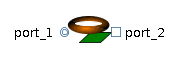Enthalpy Flow Sensor - MapleSim Help

Enthalpy Flow Sensor

Enthalpy flow sensorDescription The Enthalpy Flow Sensor component is a two-port thermal-fluid component that generates a real signal proportional to the enthalpy flow rate from ${\mathrm{flowPort}}_{a}$  to ${\mathrm{flowPort}}_{b}$.Equations $y={H}_{{\mathrm{flow}}_{a}}$ $\Delta p={p}_{a}-{p}_{b}=0$ ${Q}_{\mathrm{flow}}=0$Variables

 Name Units Description Modelica ID $\mathrm{dp}$ $\mathrm{Pa}$ Pressure drop a->b dp ${Q}_{\mathrm{flow}}$ $W$ Heat exchange with ambient Q_flowConnections

 Name Description Modelica ID ${\mathrm{flowPort}}_{a}$ flowPort_a ${\mathrm{flowPort}}_{b}$ flowPort_b $y$ Real output; enthalpy flow rate in $W$ yParameters

 Name Default Units Description Modelica ID $\mathrm{medium}$ $\mathrm{fluidMedium}$ mediumModelica Standard Library The component described in this topic is from the Modelica Standard Library. To view the original documentation, which includes author and copyright information, click here.